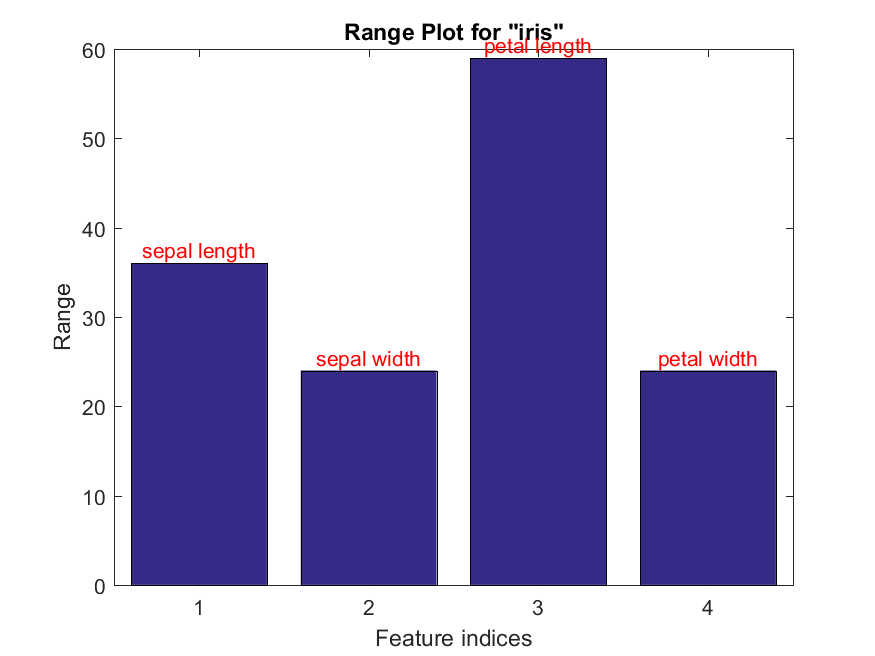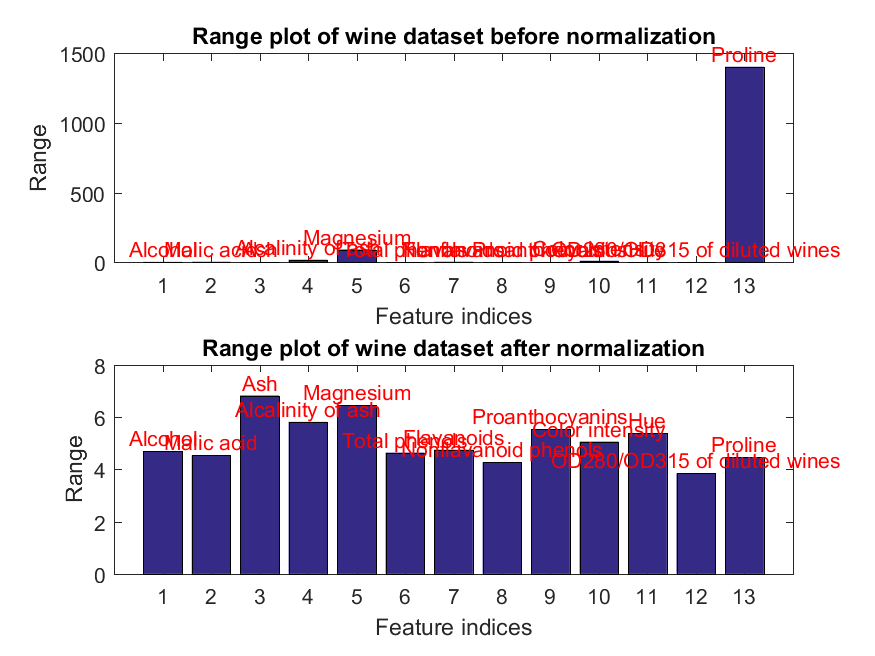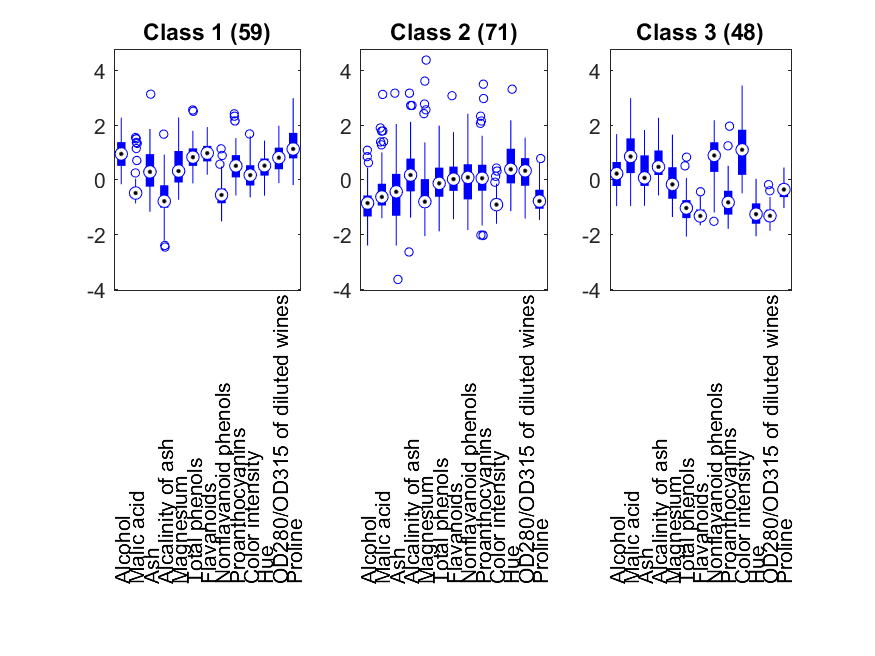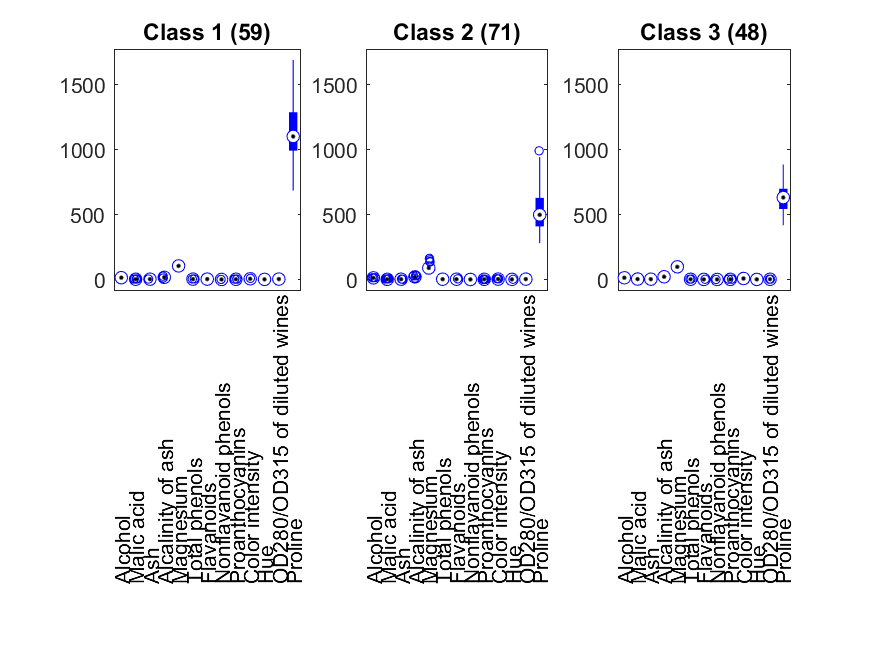## 10-1 Introduction (蝪∩?)

Old Chinese version

Before using the collected dataset, we need to pre-process the data in order to make them more suitable for constructing a classifier. Some of the commonly used pre-processing techniques are described next.

1. Data normalization
1. Conversion from nominal values to numerical values: Some datasets have features that take nominal or symbolic values instead of numerical ones. For example, the feature of gender could take "male" or "female" as the nominal values. In general, we can simply convert the nominal values into their numerical ones by taking their proximity into consideration. For instance, when converting nominal values of "sparrow", "pigeon", "turkey", and "ostrich", the result of [1 2 3 4] is better than [1 4 3 2] since the distance between "sparrow" and "ostrich" (3 in the first result, 1 in the second result) should be larger instead of smaller. Alternatively, we can also construct a classifier for each nominal value of a given feature.
2. Missing value handling: All missing values should be replaced with numerical values. A simple way to do this is to find the nearest neighbor (where the missing feature is not used for distance computation) and replace the missing value with the one of the nearest neighbor.
3. Feature normalization: Most classifiers compute the distance between two points by the Euclidean distance or the likes. If one of the features has a wide range of values, then the distance will be dominated by this feature. As a result, we should normalize the range of all features such that each feature contributes more or less equally to the final distance. In practice, there are two ways for feature-wise normalization:
• Perform a linear transformation such that the new features approximate a zero-mean unity-variance Gaussian distribution.
• Perform a linear transformation such that the new features fall within either the range of [-1, 1] or [0 1].
Note that the above feature normalization procedures are performed feature by feature. They do not take the inter-feature interaction into consideration.
2. Data reduction
1. Feature selection: To improve the recognition rate as well as reduce the computation load, we can perform feature selection by selecting the most influential features from the feature set. This will be cover in this chapter.
2. Feature extraction: To maximize the separation between different classes, we can perform feature extraction by finding a linear transformation to transform the original feature vector into a new one. This will be cover in the next chapter.
Here we shall give some examples of input (feature) normalization. First of all, we can use the function dsRangePlot.m to plot the range of each feature in a dataset. The range of a feature is defined as the maximum minus the minimum of the feature in the dataset. Moreover, we can define the range ratio as the ratio between the maximum and the minimum value of the ranges of all features. For Iris dataset, we can compute the range and the range ratio, as follows.

Example 1: rangePlot4iris01.mDS=prData('iris'); rangeVec=dsRangePlot(DS); rangeRatio=max(rangeVec)/min(rangeVec); fprintf('range ratio = %g\n', rangeRatio);range ratio = 2.45833The range ratio of the Iris dataset is around 2.46, indicating the ranges among features do not vary too much.

In contrast, the range ratio of the wine dataset is much larger, indicating that perhaps we should perform input normalization, as shown in the next example:

Example 2: rangePlot4wine01.mDS=prData('wine'); subplot(2,1,1); rangeVec1=dsRangePlot(DS); title('Range plot of wine dataset before normalization'); fprintf('range ratio before normalization = %g\n', max(rangeVec1)/min(rangeVec1)); DS.input=inputNormalize(DS.input); subplot(2,1,2); rangeVec2=dsRangePlot(DS); title('Range plot of wine dataset after normalization'); fprintf('range ratio after normalization = %g\n', max(rangeVec2)/min(rangeVec2));range ratio before normalization = 2645.28 range ratio after normalization = 1.7727In the above example, we use the function inputNormalize.m to do feature-wise zero-mean unity-variance normalization. After input normalization, range ratio is reduced from 2645.28 to 1.77. In the next section, we shall demonstrate that input (feature) normalization does improve the performance of our classifier for the Wine dataset.

A more detailed plot of the data distribution over different classes can be achieved by the command dsDistPlot.m (which requires the Statistics Toolbox):

Example 3: distPlot4wine01.mDS=prData('wine'); dsDistPlot(DS); DS.input=inputNormalize(DS.input); figure; dsDistPlot(DS);Data Clustering and Pattern Recognition (資料分群與樣式辨認)